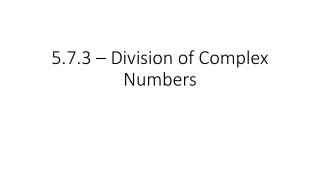DownloadDownload Presentation5.7.3 – Division of Complex Numbers

# 5.7.3 – Division of Complex Numbers

Télécharger la présentation## 5.7.3 – Division of Complex Numbers

- - - - - - - - - - - - - - - - - - - - - - - - - - - E N D - - - - - - - - - - - - - - - - - - - - - - - - - - -
##### Presentation Transcript

1. 5.7.3 – Division of Complex Numbers

2. We now know about adding, subtracting, and multiplying complex numbers • Combining like terms • Reals with reals • Imaginary with imaginary • When we multiply, treat as two binomials • FOIL • Sqaure • i2 = -1

3. Division • The division of two complex numbers is a little more challenging • Rule: You cannot have a complex number as the denominator (bottom) of a fraction • What else can’t we have in the bottom of fractions? • Luckily, there is an easy to get rid of these using a specific property

4. Rationalize • To rationalize the division of complex numbers, we will take use of the following property; • If you have the number a +bi, the complex conjugate is a – bi • Example. If you have 3 + 5i, the conjugate is 3 – 5i • Property: • (a + bi)(a – bi) = a2 – b2

5. Example. Identify the complex conjugates of the following complex numbers • A) 4 + 7i • B) -3 – 9i • C) 10i • D) 4i + 3

6. Example. Simplify the following expressions. • A) (1 + 2i)(1 – 2i) • B) (3 – 2i)(3 + 2i) • C) (6 + 2i)(6 – 2i)

7. Division of Complex Numbers • Now, from this, we can now look into how we divide complex numbers • If given the complex number 6/(5 + i), we must get rid of the complex number in the bottom • To do this, we will multiply top and bottom by the complex conjugate

8. Example. Simplify the expression 6/(5 + i) • Short cut for multiplying the complex conjugates?

9. Example. Simplify (5 – 3i)/(1 + i)

10. Example. Simplify (2 + i)/(1 – i)

11. Assignment • Pg. 265 • 51 – 63 all